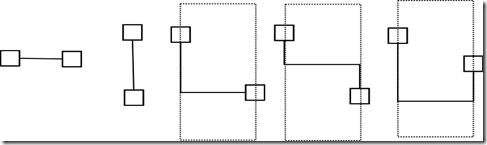# Qt版连连看之极速连连看：路线判定算法

2016/06/26 21:33``````bool
int *nPos,Pos *pos1,Pos *pos2)
{
assert(isValid(x1_,y1_));
assert(isValid(x2_,y2_));

bool posFlag=false;//传入参数中是否有折点
if(nPos){
posFlag=true;
if(!(pos1&&pos2))
return false;
}

int x1=x1_;
int x2=x2_;
int y1=y1_;
int y2=y2_;
if(!isSame(x1,y1,x2,y2))
return false;

//First,check whether they can be directly linked
if(posFlag) *nPos=0;

return true;
}
//Second,check whether they can be linked with one turning
if(findInteractPoint(x1,y1,x2,y2)){
if(posFlag){
*nPos=1;
pos1->x=x2;
pos1->y=y2;
}
return true;
}
//Third,check x-direct point that can be linked with one turning
for(int i=0;i<xMax;++i){
if(isBlank(i,y1) &&
findInteractPoint(i,y1,x2,y2)){
if(posFlag){
*nPos=2;
pos1->x=i;
pos1->y=y1;
pos2->x=x2;
pos2->y=y2;
}
return true;
}
}
//Fourth,check y-direct point that can be linked with one turning
for(int i=0;i<yMax;++i){
if(isBlank(x1,i) &&
findInteractPoint(x1,i,x2,y2)){
if(posFlag){
*nPos=2;
pos1->x=x1;
pos1->y=i;
pos2->x=x2;
pos2->y=y2;
}
return true;
}
}
return false;
}
/*
* 寻找以 (x1,y1)和 (x2,y2)所在线段为对角线的矩形中对这两个点均可直线到达的顶点
* 若找到，则将 (x2,y2)改写为该顶点，否则，返回false
*/
bool
DrawArea::findInteractPoint(const int &x1,const int &y1,int &x2,int &y2)
{
if(!board[x1][y2]){
x2=x1;
return true;
}
}
if(!board[x2][y1]){
y2=y1;
return true;
}
}
return false;
}
/*
* 检验两点是否可以直线无障碍到达
*/
bool
{
if(x1 == x2){
if(y1>y2)    std::swap(y1,y2);

for(y1+=1;y1<y2;++y1)
if(board[x1][y1])
return false;

}else if(y1 == y2){
if(x1>x2)    std::swap(x1,x2);

for(x1+=1;x1<x2;++x1)
if(board[x1][y1])
return false;
}else
return false;

return true;
}``````

``````/* 判断是否已经进入死局，死局条件：
* 在回合尚未结束的情况下遍历面板，无法找到一对可销图块
* 则判定为死局。若找到一对可销图块，则将其记录为hintA
* 和hintB,以供hint()函数利用。
*/
bool
{
int i,j,x,y;
if(!pairLeft)
return true;
for(i=0;i<xMax;++i){
for(j=0;j<yMax;++j){
if(board[i][j]){
for(x=i;x<xMax;++x){
if(x==i)    y=j+1;
else y=0;

for(;y<yMax;++y){
if(board[x][y]==board[i][j]){
hintA.x=i;
hintA.y=j;
hintB.x=x;
hintB.y=y;
return false;
}
}
}
}
}
}
}
return true;
}``````

0
0 收藏

### 作者的其它热门文章0 评论
0 收藏
0